## 一、基础操作

1.1 基本函数部分函数的笔记： Mean(): M = mean(A) 返回A的平均值，A为向量时返回所有值的平均值，A为矩阵时返回行向量，其中每一个值为该列的平均值。因此求矩阵所有元素平均值时要用两次mean()。   Imadjust(): J = imadjust(I,[low_in; high_in],[low_out; high_out],gamma) 此函数可以用于调整对比度，原理是将图片I的值映射到图片J，即把low_in到high_in的值映射到low_out上。若此参数省略，则默认使图像1%数据饱和至最低和最高亮度。 当imadjust(I,[0,1],[1,0])时取反，与imcomplement()作用相同。 参数Gamma:用于描述I与J的函数形状，省略即为线性映射。因此，可以从函数中看出，y<1 brighter, y>1 darker 注意，I要为uint8，不能为double！ Mat2gray(): I =mat2gray(A, [amin amax]) 把矩阵A转化为灰度图，将amin和amax映射到图片I的0到1，可用于增强对比度。 Imcrop(): I2 = imcrop(I,rect) 剪切图片I，rect为矩阵[xmin ymin width height]，返回剪切后的图片I2 注意事项 1.图像相加可以减少噪声，也可以用于增加曝光度（high dynamic range image） 2.图像相减可以用于运动检测 3.在图像显示的时候，要先调成uint8的类型，否则无法正常显示。注意，对图像的操作大部分基于double格式的。   1.2 色彩空间 色彩空间用数学方式来描述颜色集合。常见的3 个基本色彩模型是RGB , CMYK和YUV。在YUV 家族中，YCbCr 是在计算机系统中应用最多的成员，其应用领域很广泛，JPEG、MPEG均采用此格式。 这里只介绍YCbCr：YCbCr其中Y是指亮度分量，Cb指蓝色色度分量，而Cr指红色色度分量。人的肉眼对视频的Y分量更敏感，因此在通过对色度分量进行子采样来减 少色度分量后，肉眼将察觉不到的图像质量的变化。 处理彩色图片时，常见的方法是提取其Y分量，因Y分量包含图片大部分特征，这样能更好对其进行滤波等操作，处理完Y分量后再组合成彩色图片。 提取Y分量代码： J=rgb2ycbcr(I); K=J(:,:,1); %1是Y，2是Cb，3是Cr   1.3 灰度变换 灰度变换指逐点改变源图像像素灰度值的方法，以改善画质。 这里介绍直方图均衡： 直方图均衡化(Histogram Equalization) 又称直方图平坦化,实质上是对图像进行非线性拉伸,重新分配图像象元值,使一定灰度范围内象元值的数量大致相等。对像素个数多的灰度级进行展宽，对像素少的灰度级缩减，这样，输入图像的每一灰度级都有相同像素点数目，即输出的图像的直方图是平的。 这样,原来直方图中间的峰顶部分对比度得到增强,而两侧的谷底部分对比度降低，如果输出数据分段值较小的话,对比度会不明显。 相关函数： imhist( i ,n)： 直接显示图像i的灰度直方图（默认为255个灰度级）； n为指定灰度级显示直方图； J=histeq（I，n）: I为输入的原图像，J为直方图均衡化后得到的图像，n为均衡化后的灰度级数，默认值为64 效果：1.4 色彩平衡算法 目的： 人眼能从变化的光照环境和成像条件下获取物体表面颜色，但成像设备不具有这样的调节功能，不同的光照环境会导致采集的图像颜色与真实颜色存在一定程度的偏差，需要选择合适的颜色平衡（校正）算法，消除光照环境对颜色显现的影响。消除图像中环境光的影响，获得原始场景图像。 a.GrayWorld 算法： 对于一幅有着大量色彩变化的图像，，三个分量的平均值趋于同一灰度值。从物理意义上讲，灰色世界法假设自然界景物对于光线的平均反射的均值在总体上是个定值，这个定值近似地为“灰色” grayworld解释：grayworld代码：
function y=GrayWorld(Image)
%RGB图像为n*m*3的数组，表示尺寸为n*m个像素，其中每个像素由RGB三个分量构成。(:,:,1)表示遍历xy，提取红色分量
r=Image(:,:,1);
g=Image(:,:,2);
b=Image(:,:,3);
%取平均值，因为是二维的，故用两次mean函数
avgR = mean(mean(r));
avgG = mean(mean(g));
avgB = mean(mean(b));
avgRGB = [avgR avgG avgB];
grayValue = (avgR + avgG + avgB)/3
scaleValue = grayValue./avgRGB;

newI(:,:,1) = scaleValue(1) * r;
newI(:,:,2) = scaleValue(2) * g;
newI(:,:,3) = scaleValue(3) * b;

y=uint8(newI);


b. ScaleByMax算法 scaleByMax解释：scaleByMax代码;
function y=ScaleByMax(img)
R = img(:,:,1);
G = img(:,:,2);
B = img(:,:,3);
Rmax=max(max(R));
Gmax=max(max(G));
Bmax=max(max(B));

Cmax=min([Rmax,Gmax,Bmax]);

% calculate the pix,which larger than Cmax
Nr=sum(R(:)>=Cmax);
Ng=sum(G(:)>=Cmax);
Nb=sum(B(:)>=Cmax);

% find the largest N for Nr Ng Nb
Nmax=max([Nr,Ng,Nb]);

% for each of the find the number of element which is larger than Rmax;
for pix=Rmax:-1:0  % R
Lr=R>=pix;
nr=sum(Lr(:));
if nr>=Nmax
Rth=pix;
break
end
end

for pix=Gmax:-1:0   % G
Lr=G>=pix;
nr=sum(Lr(:));
if nr>=Nmax
Gth=pix;
break
end
end

for pix=Bmax:-1:0 % B
Lr=B>=pix;
nr=sum(Lr(:));
if nr>=Nmax
Bth=pix;
break
end
end
result(:,:,1) = Cmax/Rth*R;
result(:,:,2) = Cmax/Gth*G;
result(:,:,3) = Cmax/Bth*B;
y = result;



## 二、空间域操作

2.1 二维卷积和滤波 Conv2(): 求二维卷积 C = conv2(A,B,shape) 求AB的二维卷积，原理如图:shape用于处理边缘，有’same’’full’’valid’三种之分，以same为例：conv2(A,B,’same’)产生的结果与A的大小相同，因此，用‘same’时把卷积核放在B位置，否则出来图像和卷积核一样大。 注意，A,B以及产生的数据都是double类型，显示时需要转化成uint8 其他相关函数： Imfilter(): 线性滤波 B = imfilter(A,h) 用n维的filter h对n维矩阵A进行卷积，输出B。   medfilt2(): 二维的中值滤波 B = medfilt2(A, [m n]) 每个像素的值是其周围mn像素的中值，若省略[m n]，默认为33。 会造成图片的模糊。   Imnoise(): 添加噪声 J = imnoise(I,type,parameters) 把某种类型的噪声加在图片I上。type的类型有：‘gaussian’ ‘poisson’ 'salt & pepper’等等。Parameters取决于噪声类型，不同类型有不同的parameters，可以省略。   2.2 滤波算子 fspecial(): 函数用于产生卷积核，用于特定的滤波 h=fspecial(type,parameters) 生成特定类型的二维卷积核. type可以是以下类型: ’average’ ’gaussian’ ‘disk’ ‘log’ ‘prewitt’等等，parameter可省略。注意每种type有对应的parameters。卷积核用于对图像卷积，以突出其某种特征，或对图像本身进行处理，如钝化或锐化等。 type的类型有： 1、‘average’ h = fspecial(‘average’, hsize) 为均值滤波，参数为hsize代表模板尺寸，默认值为[3,3] Eg: 0.1111 0.1111 0.1111 0.1111 0.1111 0.1111 0.1111 0.1111 0.1111 2、 ‘disk’ circular averaging filter h = fspecial(‘disk’, radius) 为圆形区域均值滤波，参数为radius代表区域半径，默认值为5. 3、‘gaussian’ Gaussian lowpass filter h = fspecial(‘gaussian’, hsize, sigma) 为高斯低通滤波，有两个参数，hsize表示模板尺寸，默认值为[3,3]，sigma为滤波器的标准差，单位为像素，默认值为0.5. Eg: 0.0000 0.0000 0.0000 0.0000 1.0000 0.0000 0.0000 0.0000 0.0000 4、‘laplacian’ filter approximating the 2-D Laplacian operator h = fspecial(‘laplacian’, alpha) 为拉普拉斯算子，用于边缘增强。参数alpha用于控制算子形状，取值范围为[0,1]，默认值为0.2. Eg: 0.3333 0.3333 0.3333 0.3333 -2.6667 0.3333 0.3333 0.3333 0.3333 5、‘log’ Laplacian of Gaussian filter h = fspecial(‘log’, hsize, sigma) 为拉普拉斯高斯算子，有两个参数，hsize表示模板尺寸，默认值为[3,3]，sigma为滤波器的标准差，单位为像素，默认值为0.5. 6、‘motion’ h = fspecial(‘motion’, len, theta) motion filter为运动模糊算子，有两个参数，表示摄像物体逆时针方向以theta角度运动了len个像素，len的默认值为9，theta的默认值为0； 7、‘prewitt’ Prewitt horizontal edge-emphasizing filter 用于水平边缘增强，大小为[3,3]，无参数，eg:[1 1 1;0 0 0;-1 -1 -1]. (注：若卷积核用于突出水平边缘特征（sober,prewitt），那么转置后便可突出垂直边缘特征，转置用transpose函数) 8、‘sobel’ Sobel horizontal edge-emphasizing filter 用于水平边缘提取，无参数，eg:[1 2 1;0 0 0;-1 -2 -1]. 9、‘unsharp’ unsharp contrast enhancement filter 为对比度增强滤波器。参数alpha用于控制滤波器的形状，范围为[3,3]，默认值为0.2.   2.3 非局部均值去噪滤波：Non-Local Means图像的形象表示：通过搜索框y，寻找相似的部分，算出权重，相加，得到x。实现代码：
function [output]=simple_nlm(input,t,f,h1,h2,selfsim)
[m,n]=size(input);
pixels = input(:);
s = m*n;
psize = 2*f+1;
nsize = 2*t+1;
% Compute patches
filter = fspecial('gaussian',psize,h1);
patches = repmat(sqrt(filter(:))',[s 1]) .* im2col(padInput, [psize psize], 'sliding')';
% Compute list of edges (pixel pairs within the same search window)
indexes = reshape(1:s, m, n);
neighbors = im2col(padIndexes, [nsize, nsize], 'sliding');
TT = repmat(1:s, [nsize^2 1]);
edges = [TT(:) neighbors(:)];
RR = find(TT(:) >= neighbors(:));
edges(RR, :) = [];
% Compute weight matrix (using weighted Euclidean distance)
diff = patches(edges(:,1), :) - patches(edges(:,2), :);
V = exp(-sum(diff.*diff,2)/h2^2);
W = sparse(edges(:,1), edges(:,2), V, s, s);
% Make matrix symetric and set diagonal elements
if selfsim > 0
W = W + W' + selfsim*speye(s);
else
maxv = max(W,[],2);
W = W + W' + spdiags(maxv, 0, s, s);
end
% Normalize weights
W = spdiags(1./sum(W,2), 0, s, s)*W;
% Compute denoised image
output = W*pixels;
output = reshape(output, m , n);



## 三、频域操作

3.1 离散傅里叶变换：DFT 相关函数： fft2(): 二维傅里叶变换 Y = fft2(X,m,n) pads X with zeros to create an m-by-n array before doing the transform. The result is m-by-n. Y=fft2(X) returns the two-dimensional discrete Fourier transform (DFT) of X, if size(X) = [100 100 3], then fft2 computes the DFT of X(:,:,1), X(:,:,2) and X(:,:,3)   频谱图：在频域中，频率越大说明原始信号变化速度越快；频率越小说明原始信号越平缓。当频率为0时，表示直流信号，没有变化。因此，频率的大小反应了信号的变化快慢。高频分量解释信号的突变部分，而低频分量决定信号的整体形象。 对图像而言，图像的边缘部分是突变部分，变化较快，因此反应在频域上是高频分量；图像的噪声大部分情况下是高频部分；图像平缓变化部分则为低频分量。 频域滤波即用高通，低通，带阻滤波器等进行滤波，以保留特定的频率成分。 对于傅里叶变换的频谱：频谱居中后，中心位置应该是最亮的，因为直流分量很大，中间低频部分的能量占据了大部分。   3.1.1高通滤波 高通滤波器可以增强图像边缘，起到锐化图像的作用。这里写理想高通滤波。 理想高通滤波器只需1减理想低通滤波器即可：滤波效果：3.1.2 低通滤波 低通滤波有多种滤波器，这里写理想低通滤波器和巴特沃斯滤波器。低通滤波器滤除了高频分量，去除了噪声，但同时一些边缘和细节也被滤除，图像边界变模糊，有平滑图像的作用。 理想低通滤波器：（其中，D0表示通带半径，D(u，v)是到频谱中心的距离）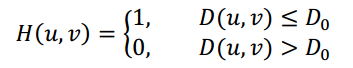例子：理想低通滤波器会造成比较严重的模糊和振铃现象，因矩形信号频谱为Sa函数，相乘后会造成无限的振铃现象。 巴特沃斯（butterworth）低通滤波器：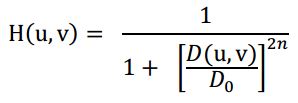从函数图上看，比理想低通滤波器更圆滑，用幂系数n可以改变滤波器的形状。n越大，则该滤波器越接近于理想滤波器 例子：可以看出butterworth低通滤波器没有严重的振铃现象。 注意事项： 矩阵点乘时特别注意两个矩阵大小相同，类型一致！ 频域相乘时，若矩阵的大小不匹配，要补0，补0的位置要选在高频位置！ Imshow时检查矩阵是否为double类型，要转化为uint8才能正常输出。   3.2 离散余弦变换：DCT DCT变换公式：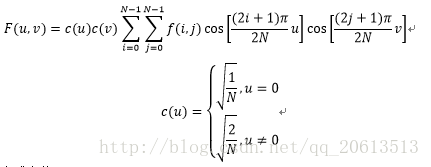DCT优势： 1.The most important reason is much reduced sensitivity to boundaries of finite sequences（DFT变换没有考虑到子图之间的边界，波形不连续容易导致Gibbs phenomenon；而DCT得到子图间的波形则是连续的。） 2 Resulting Compaction（可压缩） 3 No complex numbers（无复数，因只取实部进行变换，实偶函数傅里叶变换也是实偶函数） 4 Number of coefficients is equal to number of pixels（变换后一个像素对应一个值） 5 DCT coefficients occur at every half cycle so Nyquist can be stated as number of coefficients should not exceed number of sample points   3.2.1 DCT域滤波 对二维灰度图像进行DCT变换，就能得到图像的频谱图：低阶(变化幅度小)的部分反映在DCT的左上方，能量也主要集中在左上角。高阶(变化幅度大)的部分反映在DCT的右下方。由于人眼对高阶部分不敏感，依靠低阶部分就能基本识别出图像内容，所以JPEG进行压缩的时候，基本上只存储DCT变换后的左上部分，而右下部分则直接丢弃。 图像转化为dct域后，其高频成分在矩阵的右下方，低频成分在左上方。可将其提取出来进行滤波。 举例说明：用3D图显示时可以看到，添加高斯噪声后，其高频位置明显大了很多，而低频变化小：附上3D图显示的函数： Meshgrid(): [X,Y] = meshgrid(x,y) [X,Y,Z] = meshgrid(x,y,z) 创造2D或3D的网格图，x和y是取值范围，如：x=x1:dx:x2，返回二维或三维的矩阵。 Surface(): surface(X,Y,Z) 创造一个曲面，x，y为范围，可以用meshgrid定义，这样会显示一个网格曲面图，上面的颜色代表Z的大小，Z越大颜色越深。 注意，x和y是向量时, length(X) = n and length(Y) = m, 那么[m,n] = size(Z)，否则会报错，向量维数不匹配。 View(): view(az,el) 设定看一个三维图的角度，az为水平转向角度，正值时顺时针转，el为垂直转向角度，正值时往屏幕方向转。如：view(60,45)Surf(): surf(X,Y,Z) 与surface相同，生成的三维图默认从(45,45)角观看 注意值的大小，太小或者太大时，可以用log函数处理其绝对值，再显示   3.2.2维纳滤波 这一种基于最小均方误差准则、对平稳过程的最优估计器。这种滤波器的输出与期望输出之间的均方误差为最小。它能根据图像的局部方差调整滤波器的输出，局部方差最大，滤波器的平滑作用就越强。 公式：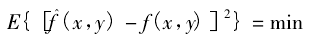其中^f为我们维纳滤波后的图像，f为清晰的原始图像 求^f的公式：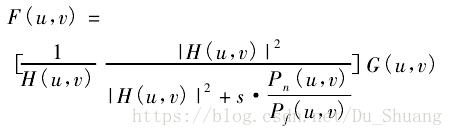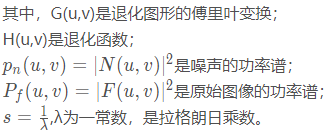维纳滤波需要知道原始图像和噪声的二阶统计特性，即要知道关于图像和噪声的功率谱，但是这恰恰也是我们不知道的，这也是维纳滤波器的局限所在，我们一般将上述两个公式的比值看做是常数带入进行计算，如下所示：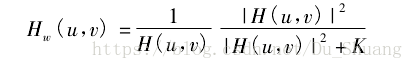这是一种粗糙的近似，但是当噪声为白噪声即其功率谱为常数的时候，这种近似效果很不错。 MATLAB对维纳滤波算法的解释：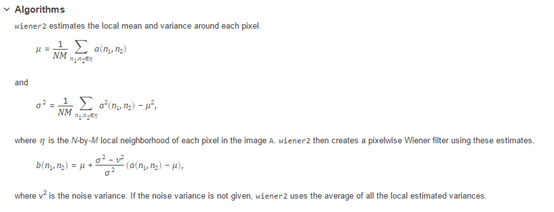实现代码：
sigma = d_im1_Y(100:end,100:end).*d_im1_Y(100:end,100:end);%d_im1_Y为图片im1的Y分量的dct，对dct变换矩阵的右下部分进行滤波，
NoiseVariance = mean(mean(sigma));%算均值，评估噪声强度，需要多次试验得出最佳系数
beta = 4.0;%与噪声移除有关的系数，越高噪声滤除越多，但图片也越模糊
NoiseVariance = beta*NoiseVariance;
SignalVariance = d_im1_Y.*d_im1_Y + 0.001;%
WienerFilter = 1 + (NoiseVariance./SignalVariance);
WienerFilter = 1./WienerFilter;
FilteredImageDCT = d_im1_Y.*WienerFilter;
FilteredImage = idct2(FilteredImageDCT);
imo = uint8(FilteredImage);
imshow(imo);


维纳滤波matlab函数： wiener2(): J = wiener2(I,[m n],noise) 对区域为m*n像素的区域作为每个像素点的neighborhood进行维纳滤波，noise为对噪声大小的评估,noise值越大，噪声去除越多，但图片也更模糊。返回滤波后的图像J。 [J,noise] = wiener2(I,[m n]) 返回滤波后的图像，以及根据图像评估的噪声强度。   J=deconvwnr（I,PSF,NSR）：维纳滤波图像复原 PSF为点扩散函数，可以由fspecial函数创建（比如PSF = fspecial(‘motion’);）， PSF与原始图像进行卷积，可得到退化（模糊）的图像。用于复原由于PSF以及可能的加性噪声卷积退化的图像I，该算法利用图像和噪声的相关矩阵，从估计图像与真实图像之间的最小均方误差。在没有噪声的情况下，维纳滤波器退化成理想的逆滤波器。NSR是信噪功率比，NSR可以是标量，或者是和图像I一样大小尺寸的数组，NSR的默认值为0。 信噪比的设置需要很准确，比如对一幅模糊和噪声的图像去模糊，中间图NSR设为0，右边图片NSR为0.005左右3.2.3 DCT域对比增强 优势：跟直方图均衡比较时，直方图均衡会破坏背景，比如本来背景不含信息，为全黑，均衡后变为灰色。而在频域操作就不会有这个问题。 代码：
im = imread('moon.tif');
imd = dct2(im);
dc = imd(1,1);
imd=imd*1.4;%乘一个比例系数，越大对比度越明显
imd(1,1)=dc;%保持直流分量不变
im2=idct2(imd);
%%%%%%%%%%%%%%%%%%减一个比例系数x
a= min(min(im2))
b= max(max(im2))-255;
x = max(a,b)

figure
imshow(im);
figure
imshow(uint8(im2-x));


3.2.4 DCT域有损压缩：JPEG 步骤： 1、将原始图像分为 8 * 8 的小块, 每个 block 里有 64 个像素。   2、 将图像中每个 8 * 8 的 block 进行 DCT 变换。8 * 8 的图象经过 DCT 变换后,其低频分量都集中在左上角,高频分量分布在右下角。由于该低频分量包含了图象的主要信息(如亮度),而高频与之相比,就不那么重要了,所以我们可以忽略高频分量。   3、 利用量化表抑制高频变量。量化操作,就是将某一个值除以量化表中对应的值。由 于量化表左上角的值较小,右上角的值较大,这样就起到了保持低频分量,抑制高 频分量的目的。舍弃高频系数（AC系数），保留低频信息（DC系数）。高频系数一般保存的是图像的边界、纹理信息，低频信息主要是保存的图像中平坦区域信息。图像的低频和高频，高频区域指的是空域图像中突变程度大的区域（比如目标边界区域），通常的纹理丰富区域。   4.压缩时候将彩色图像的颜色空间由 RGB 转化为 YUV 格式。其中 Y 分量代表了亮度信息,UV 分量代表了色差信息。相比而言,Y 分量更重要一些。 我们可以对 Y 采用细量化,对 UV 采用粗量化,可进一步提高压缩比。所以量化表 通常有两张,一张是针对 Y 的标准亮度量化表;一张是针对 UV 的标准色彩量化表。   5.经过量化之后右下角大部分数据变成了 0,左上角为非零数据。这时使用 Z 字型(如 图所示)的顺序来重新排列数据生成一个整数数组,这样 0 就位于数组都后端。找到数组最后一个非零元素,将其后的数据都舍弃,并加上结束标志。   示意图：对图片中一个小块作Dct，高频部分去除之后，图片有些模糊，去除的越多，图像变化越大。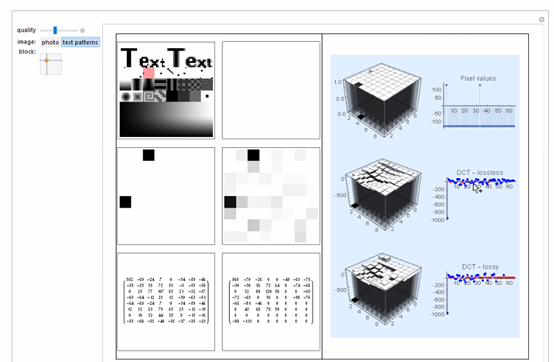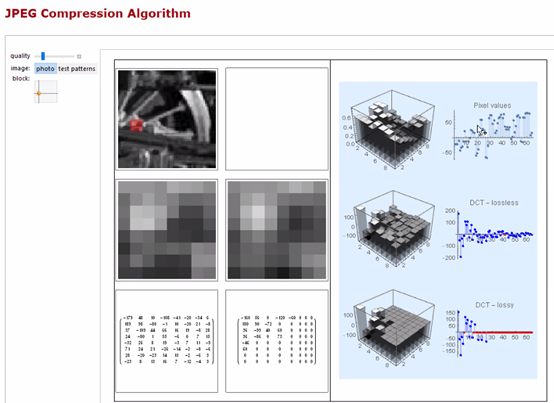图像压缩编码：Huffman编码 优点： 根据符号出现频率决定编码长度，频率高的出现多，信息量少，可以节约空间。而频率低的符号信息量大，因此编码更长。 做法： 统计每个符号出现的概率，从小到大排列。而后每次选出两个最小的值作为二叉树的节点，他们的和作为根节点。 示意图: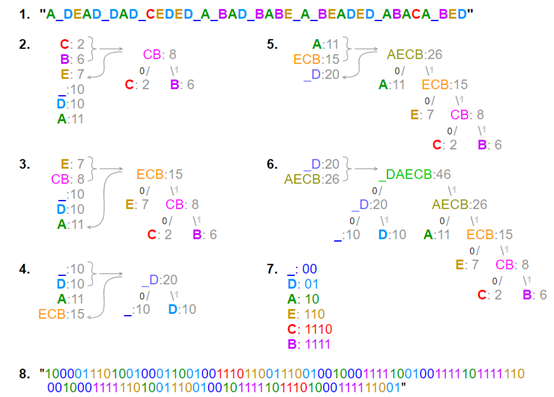## 4.图像分割

figure, imshow(image), hold on

max_len = 0;
for k = 1:length(lines)
xy = [lines(k).point1; lines(k).point2];
plot(xy(:,1),xy(:,2),'LineWidth',2,'Color','green');

% Plot beginnings and ends of lines
plot(xy(1,1),xy(1,2),'x','LineWidth',2,'Color','yellow');
plot(xy(2,1),xy(2,2),'x','LineWidth',2,'Color','red');

% Determine the endpoints of the longest line segment
len = norm(lines(k).point1 - lines(k).point2);
if ( len > max_len)
max_len = len;
xy_long = xy;
end
end
% highlight the longest line segment
plot(xy_long(:,1),xy_long(:,2),'LineWidth',2,'Color','red');